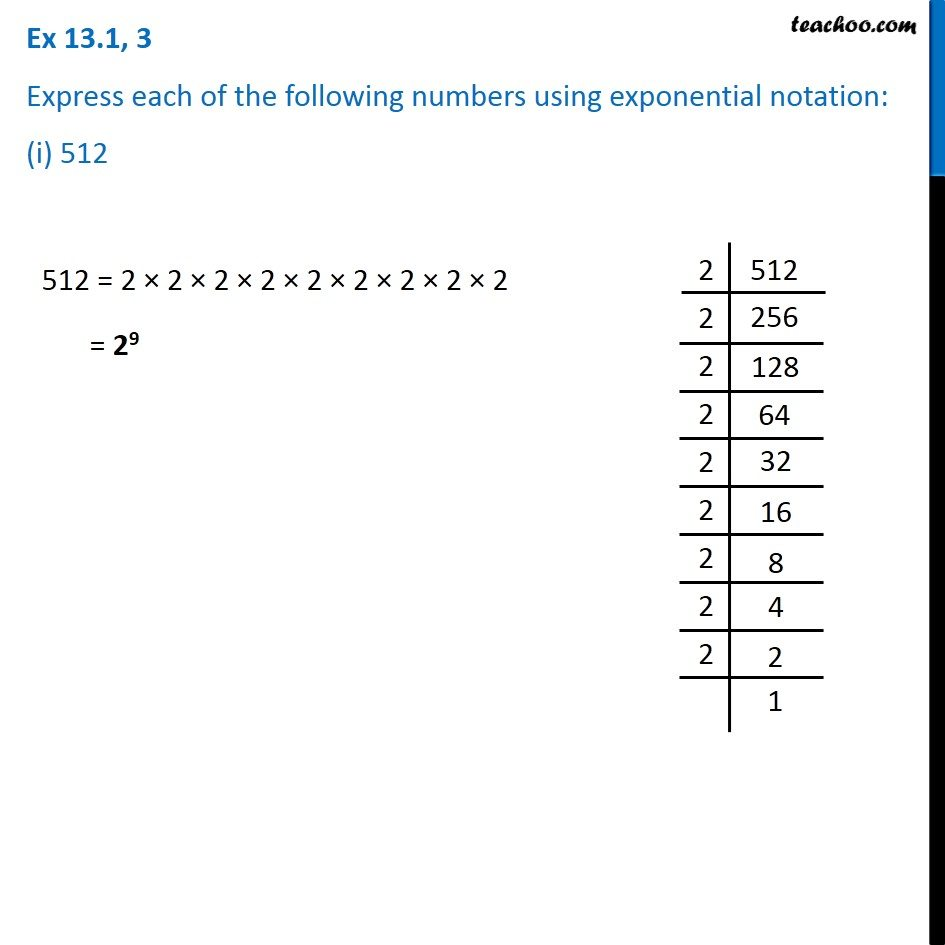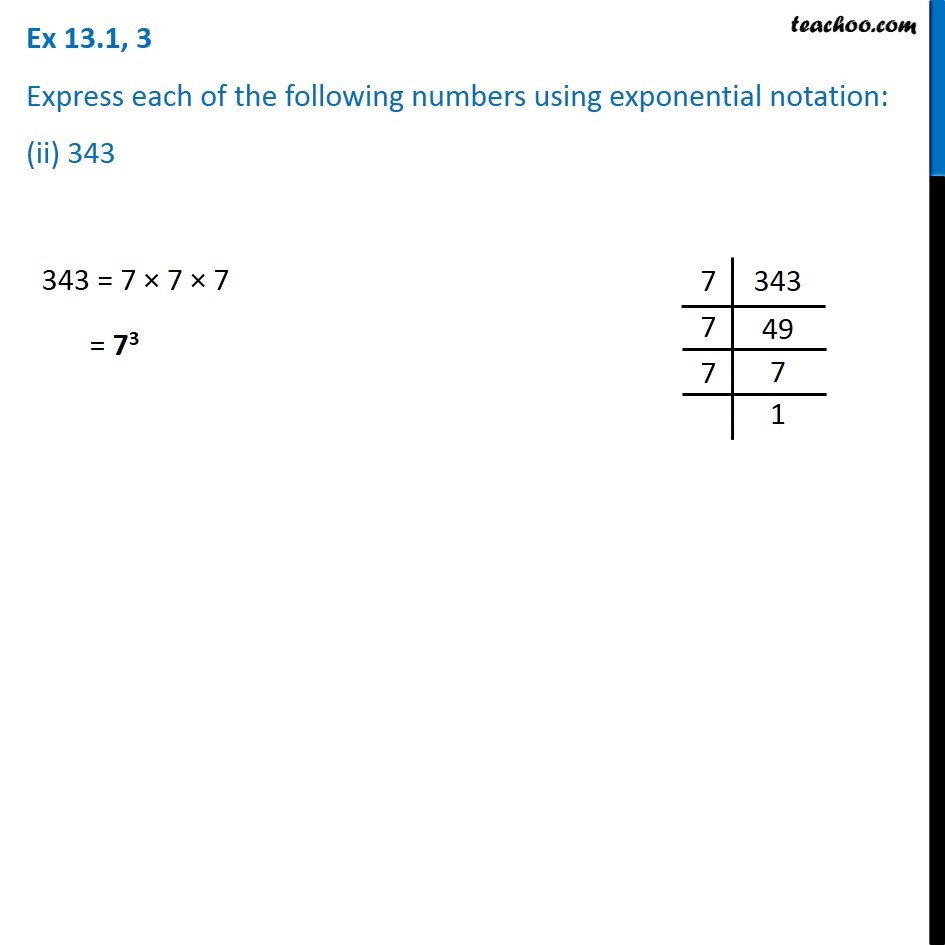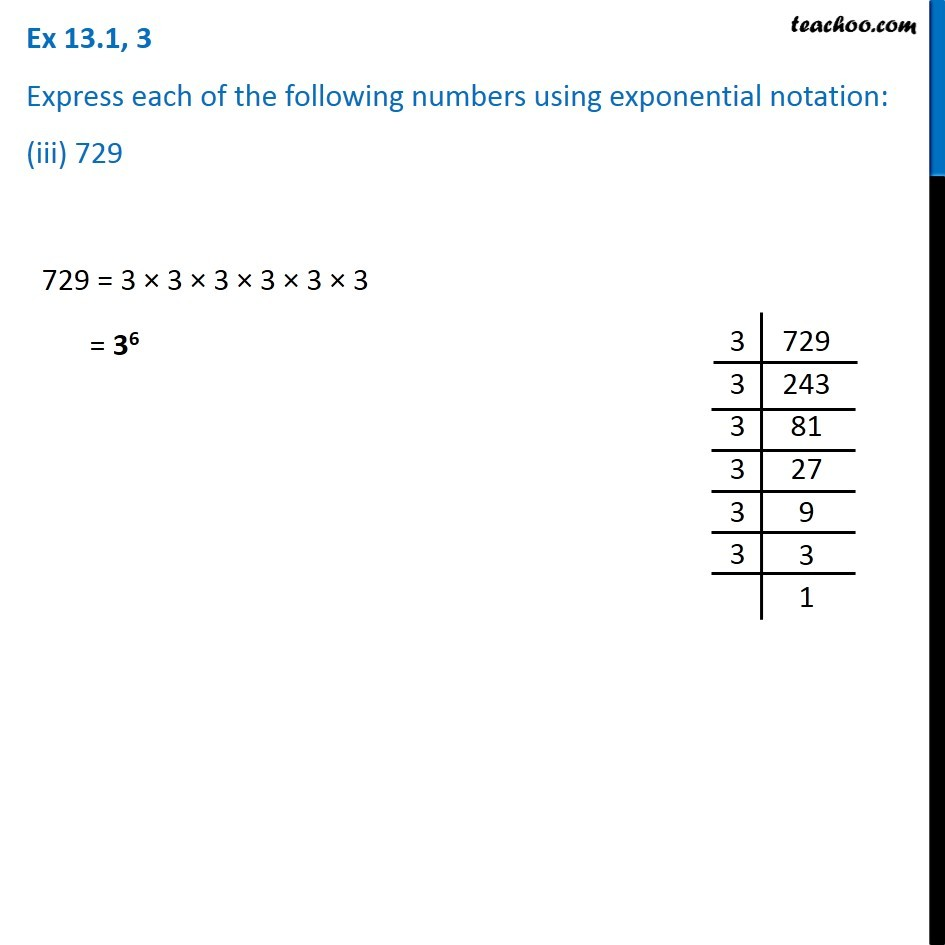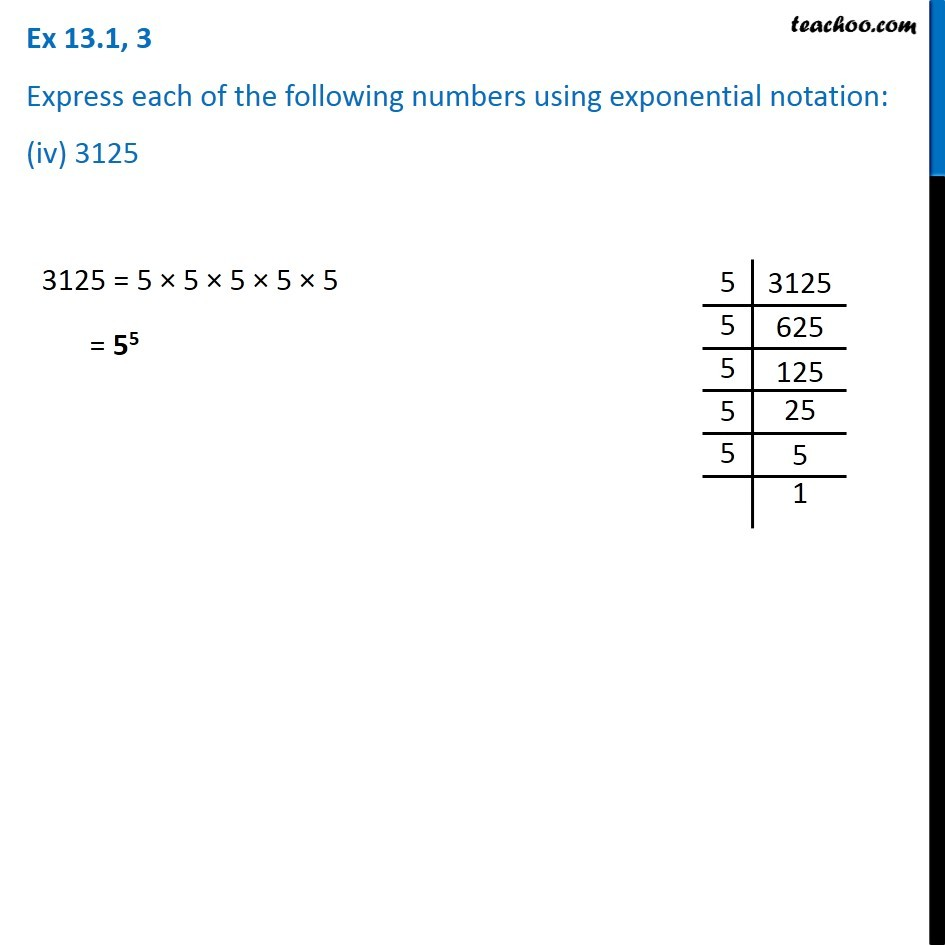Subscribe to our Youtube Channel - https://you.tube/teachoo

1. Chapter 13 Class 7 Exponents and Powers
2. Serial order wise
3. Ex 13.1

Transcript

Ex 13.1, 3 Express eac.h of the following numbers using exponential notation: (i) 512 512 = 2 × 2 × 2 × 2 × 2 × 2 × 2 × 2 × 2 = 29 Ex 13.1, 3 Express each of the following numbers using exponential notation: (ii) 343 343 = 7 × 7 × 7 = 73 Ex 13.1, 3 Express each of the following numbers using exponential notation: (iii) 729 729 = 3 × 3 × 3 × 3 × 3 × 3 = 36 Ex 13.1, 3 Express each of the following numbers using exponential notation: (iv) 3125 3125 = 5 × 5 × 5 × 5 × 5 = 55

Ex 13.1